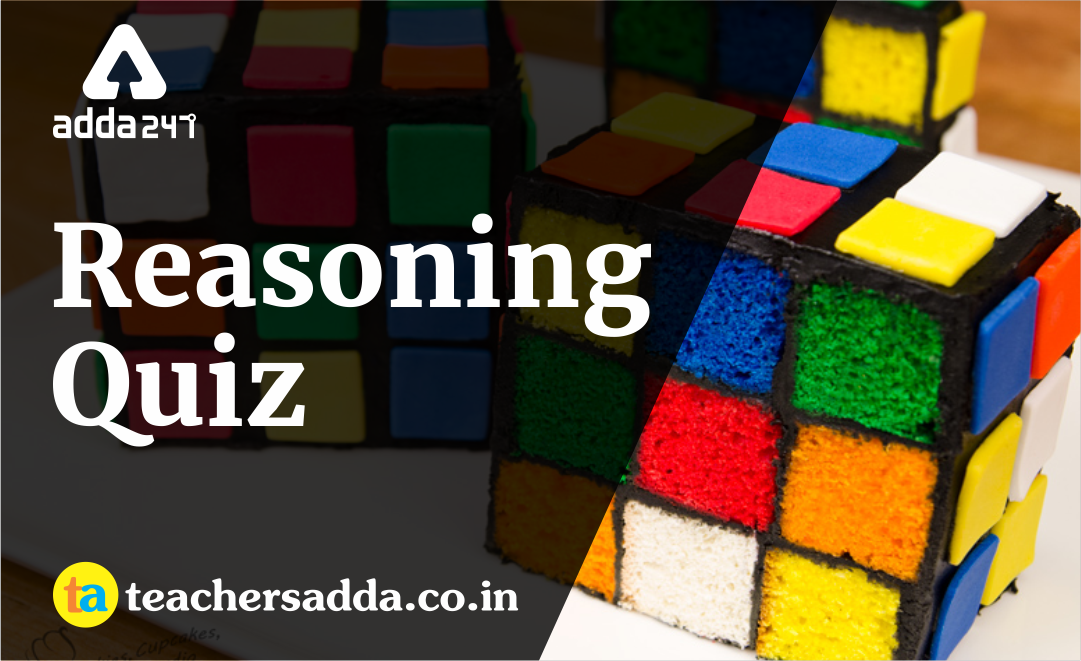The reasoning is the most scoring section in any competitive exam. It also develops or nourishes our critical thinking skills as compared to other subjects. In DSSSBKVSAPS Exam as a student to score well in this part, you have to start your preparation now because there is negative marking as well. The more you have prepared, the better you score.

Q1. The next term in the series
2, 7, 28, 63, 126, ____ is
(a) 215
(b) 245
(c) 276
(d) 296

Q2. The next term in the series
AB, ED, IH, NM, _____ is
(a) TS
(b) ST
(c) TU
(d) SU

Q3. If STREAMERS is coded as UVTGALDQR, then KNOWLEDGE will be coded as
(a) MQPYLCDFD
(b) MPQYLDCFD
(c) PMYQLDFCD
(d) YMQPLDDFC

Q4. Two numbers are in the ratio 3: 5. If 9 are subtracted from the numbers, the ratio becomes 12: 23. The numbers are
(a) 30, 50
(b) 36, 60
(c) 33, 55
(d) 42, 70

Q5. Assertion (A): Formal communication tends to be fast and flexible.
Reason(R): Formal communication is a systematic and orderly flow of information.
(a) Both (A) and (R) are correct, and (r) is the correct explanation of (A).
(b) Both (A) and (R) are correct, but (R) is not the correct explanation of (A).
(c) (A) is correct, but (R) is false
(d) (A) is false, but (R) is correct.

Q6. The next term in the series is
2, 5, 9, 19, 37,?
(a) 72
(b) 75
(c) 78
(d) 80

Q7. In certain code, MATHURA is coded as JXQEROX. The code of HOTELS will be
(a) LEQIBP
(b) ELQBIP
(c) LEBIQP
(d) ELIPQB

Q8. One day Prakash left home and walked 10 km towards south, turned right and walked 5 km, turned right and walked 10 km and turned left and walked 10 km. how many km will he have to walk to reach his home straight?
(a) 10
(b) 20
(c) 15
(d) 30

Q9. A girl introduced a boy as the son of the daughter of the father of her uncle. The boy is related to the girl as
(a) Brother
(b) Uncle
(c) Nephew
(d) Son

Q10. At present, a person is 4 times older than his son and is 3 years older than his wife. After 3 years, the age of the son will be 15 years. The age of the person’s wife after 5 years will be
(a) 42
(b) 48
(c) 45
(d) 50

Solutions

S1. Ans.(a)
Sol.
1³ + 1, 2³ – 1, 3³ + 1, 4³ – 1, 5³ + 1 and so on. Hence, the answer is 215.

S2. Ans.(a)
Sol.
B + 2 gives D, D + 1(and reversing order) gives ED, again D + 4 gives H and so on. Hence, option (a) is the answer.

S3. Ans.(b)
Sol.S4. Ans.(C)
Sol.
Let’s assume that numbers are 3x and 5x.
According to the statement, (3x–9)/ (5x–9) = 12/33 solving it we get x = 11. Hence, the numbers are 33 and 55.

S5. Ans.(d)
Sol.(A) is false but, (R) is correct

S6. Ans.(b)
Sol.
x2 + 1, x2 – 1, x2 + 1 and so on.
2x2 + 1, 5x2 – 1, 9x2 + 1, 19x2 – 1, and so on.

S7. Ans.(b)
Sol. The coding is 3rd letter backwards so HOTELS will become ELQBIP.

S8. Ans.(b)
Sol.S9. Ans.(a)
Sol. Daughter of uncle's father — Uncle's sister — Mother;
Mother's son — Brother

S10. Ans.(d)
Sol.
Let us assume that present age of person = x years.
Hence, son’s age = x/4 years.
According to the statement x/4 = 15 – 3 = 12 years
⇒ x = 48 years.
Hence, wife’s age = 48 – 3 = 45 years.
Wife’s age after 5 years = 45 + 5 = 50 years.

You may also like to read :